EXERCISE 5.1

1) Find the complement of each of the following angles:
Ans: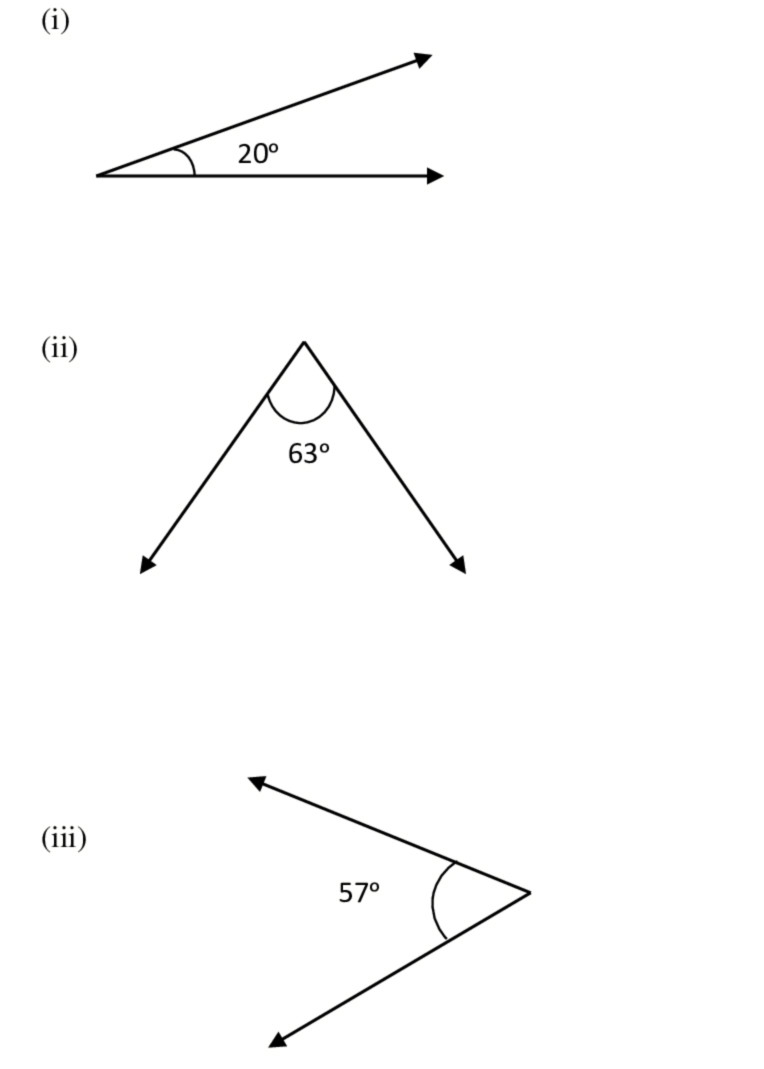i)The given angle is 20ᵒ
Let its complement be x
Therefore, x + 20ᵒ = 90ᵒ
x = 90ᵒ − 20ᵒ
x = 70ᵒ
ii)The given angle is 63ᵒ
Let its complement be x
Therefore, x + 63ᵒ = 90ᵒ
x = 90ᵒ − 63ᵒ
x = 27ᵒ
iii)The given angle is 57ᵒ
Let its complement be x
Therefore, x + 57ᵒ = 90ᵒ
x = 90ᵒ − 57ᵒ
x = 33ᵒ

2) Find the supplement of each of the following angles:
Ans: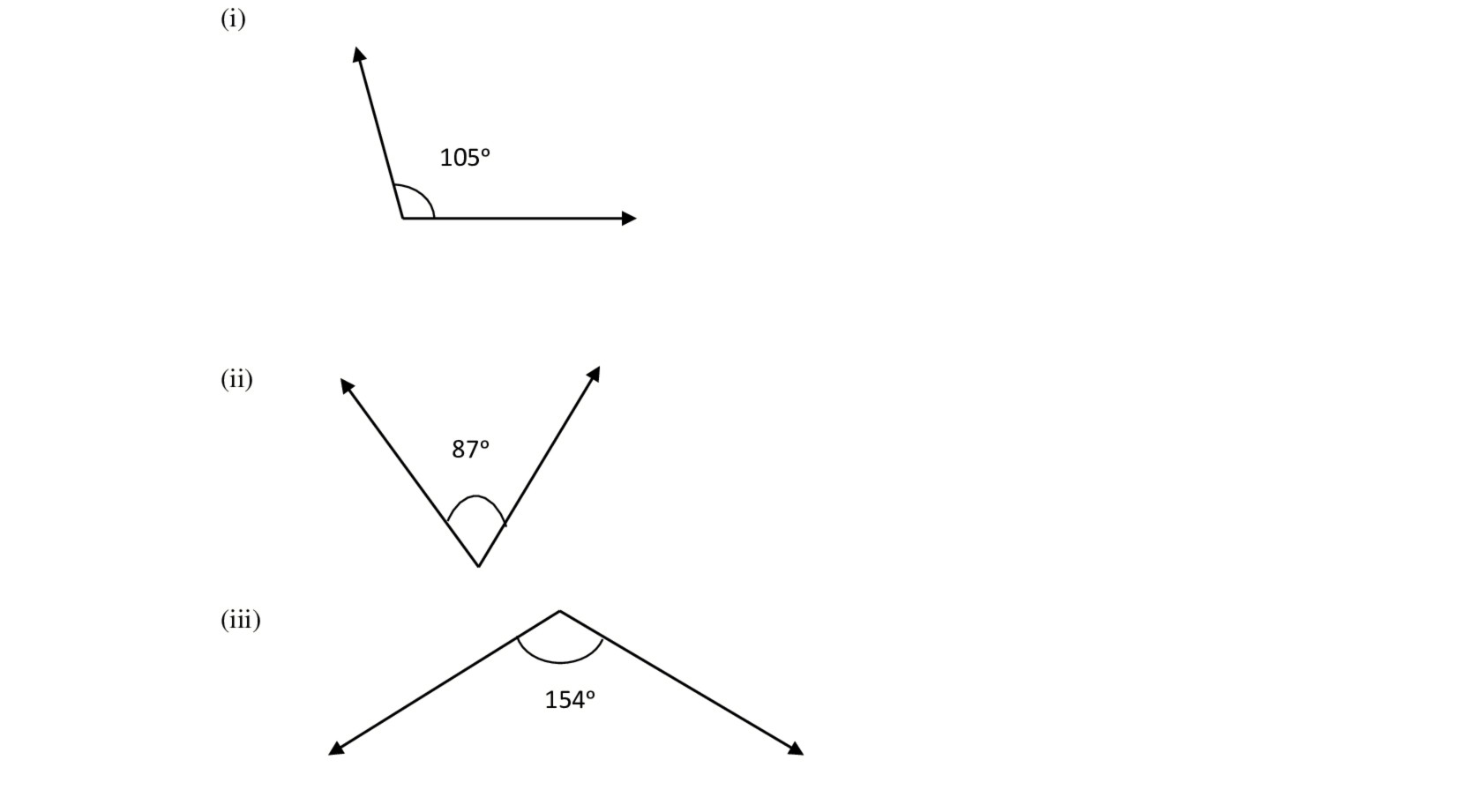i)The given angle is 105ᵒ
Let its supplement be xᵒ
x + 105ᵒ = 180ᵒ   → x = 180ᵒ − 105ᵒ   → x = 75ᵒ
ii)The given angle is 87ᵒ
Let its supplement be xᵒ
x + 87ᵒ = 180ᵒ   → x = 180ᵒ − 87ᵒ   → x = 93ᵒ
iii)The given angle is 154ᵒ
Let the supplement be xᵒ
x + 154ᵒ = 180ᵒ   → x = 180ᵒ − 154ᵒ  → x = 26ᵒ

3). Identify which of the following pairs of angles are complementary and which are supplementary :
65ᵒ , 115ᵒ
Ans:
i) 65ᵒ + 115ᵒ

= 180ᵒ
Therefore, these angles are supplementary.
ii) 63ᵒ , 27ᵒ

Ans: 63ᵒ + 27ᵒ
= 90ᵒ
Therefore, these angles are complementary.
iii)112ᵒ, 68ᵒ
Ans: 112ᵒ + 68ᵒ
= 180ᵒ
Therefore, the angles are supplementary.
iv) 130ᵒ , 50ᵒ

Ans: 130ᵒ + 50ᵒ
= 180ᵒ
Therefore, these angles are supplementary.
v) 45ᵒ, 45ᵒ
Ans: : 45ᵒ + 45ᵒ = 90ᵒ
Therefore, these angles are complementary.

4) Find the angle which is equal to its complement.
Ans: Let the measure of the required angle be x.
Since the sum of the complementary angle is 90ᵒ.
x + x = 90ᵒ
2 x = 90ᵒ
x = 90ᵒ/2
x = 45ᵒ
Hence the required angle measure is 45ᵒ.

5)Find the angle which is equal to its supplement.

Ans: Let the measure of the required angle be x
We know that sum of measure of supplementary angle is 180ᵒ.
Therefore, x + x = 180ᵒ
2 x = 180ᵒ
x = 180ᵒ/2
x = 90ᵒ
Hence, the required angle measure is 90ᵒ.

6)In the given, ∠1 and ∠2 are supplementary. If ∠1 is decreased, what changes should take place in ∠2 so that both the angles still remain supplementary
Ans: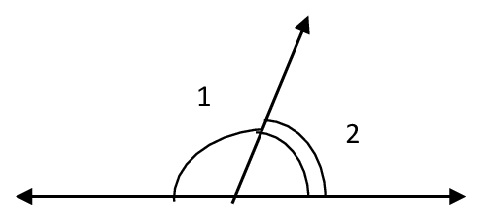It is given that ∠1 and ∠2 are supplementary

If ∠1 is decreased, then ∠2 must be increased by the same value.
Hence, this angle pair remains supplementary.

7) Can two angles be supplementary if both of both them are:
i) Acute?
Ans: No. If two angles are acute, they cannot be supplementary as each angle is less than 90ᵒ and hence their sum wont be 180ᵒ.
ii) Obtuse?

Ans: No. If two angles are obtuse, then each angle measure is more than 90ᵒ and so their sum will be always more than 180ᵒ.
iii) Right?

Ans: Yes. If two angles are right, it means that their sum will be 180ᵒ as measure of each right angle is 90ᵒ.

8) An angle is greater than 45ᵒ. Is its complementary angle greater than 45ᵒ or equal to 45ᵒ or less than 45ᵒ?
Ans: Let us assume the complementary angles to be a and b
We know that the sum of measures of complementary angle pair is 90ᵒ
Then a + b = 90ᵒ
It is given that a > 45ᵒ
= a + b > 45ᵒ + b
= 90ᵒ > 45ᵒ + b
= 90ᵒ − 45ᵒ > b
= b < 45ᵒ
Hence, its complementary angle is less than 45ᵒ.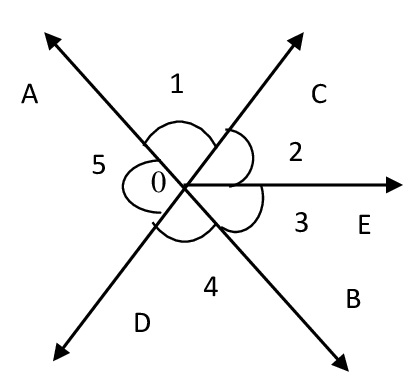i) Is ∠1 adjacent to ∠2?
Ans: Yes.
ii) Is ∠AOC adjacent to ∠AOE?
Ans: No. 0
iii) Do ∠COE and ∠CEO form a linear pair?
Ans: Yes.
iv) Are ∠BOD and ∠DOA supplementary?
Ans: Yes.
v) Is ∠1 vertically opposite to ∠4?
Ans: Yes.
vi) What is the vertically opposite angle of ∠5?
Ans: ∠COB.

10) Indicate which pairs of angles are: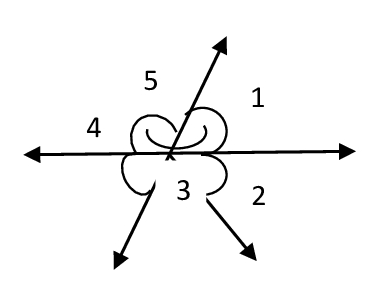(i) Vertically opposite angles
Ans: ∠1 and ∠4 , ∠5   and    ∠2+ ∠
(ii) Linear pairs
Ans: ∠1 and ∠5   and   ∠5 and ∠4

11) In the following figure, is ∠1 adjacent to ∠2 ? Give reasons.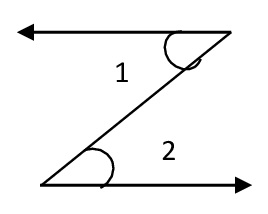Ans: No, ∠1 and ∠2 are not adjacent angles because they do not have a
common vertex.

12) Find the values of the angles x, y and z in each of the following:
Ans:i) ∠y + 55ᵒ = 180ᵒ —- Linear pair
∠y = 180ᵒ − 55ᵒ
∠y = 125ᵒ
∠x = 55ᵒ —– vertically opposite angles
∠y = ∠x —— vertically opposite angles
.’ . ∠z = 125ᵒii) ∠40ᵒ + ∠x + 25ᵒ = 180ᵒ —- angles on a straight line
∠x = 180ᵒ − 65ᵒ
∠x = 115ᵒ
∠z = 40ᵒ —— vertically opposite angles
∠y + ∠z = 180ᵒ
∠y = 180ᵒ − ∠z
= 180ᵒ − 40ᵒ
= 140ᵒ

13) Fill in the blanks:
i) If two angles are complementary, then the sum of their measures is _________.
ii) If two angles are supplementary, then the sum of their measures is __________.
iii) Two angles forming a linear pair are ________.
iv) If two adjacent angles are supplementary, they form a ________.
v) If two lines intersect at a point, then the vertically opposite angles are always ________.
vi) If two lines intersect at a point, and if one pair of vertically opposite angles are acute angles, then the other pair of vertically opposite angles are ________.
Ans:
i) 90ᵒ
ii) 180ᵒ
iii) Supplementary
iv) Linear pair
v) Equal
vi) Obtuse angle

14) In the adjoining figure, name the following pairs of angles: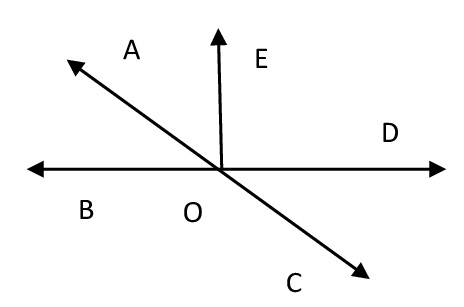i) Obtuse vertically opposite angles
Ans: ∠AOD and ∠BOC